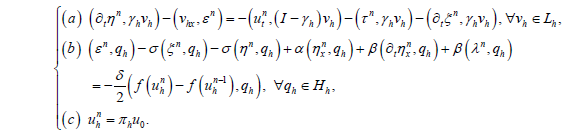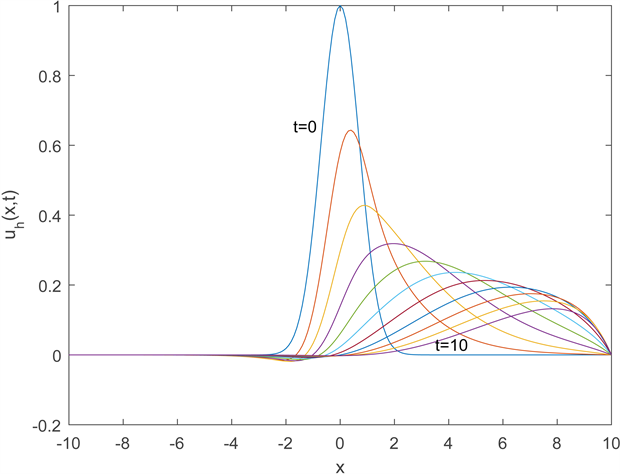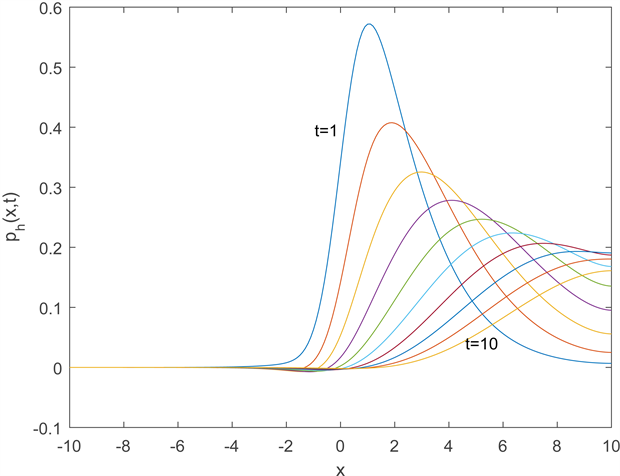正则长波Burgers方程的混合有限体积元方法Mixed Finite Volume Element Method for Regularized Long Wave Burgers Equation

• 全文下载: PDF(836KB)    PP.257-268   DOI: 10.12677/AAM.2018.73032
• 下载量: 493  浏览量: 641   国家自然科学基金支持

The mixed finite volume element method for the regularized long wave Burgers equation is de-veloped and studied. By introducing a transfer operator which maps the trial function space into the test function space, the semi-discrete and linear backward Euler fully discrete mixed finite volume element schemes are constructed. Stability analysis for semi-discrete scheme is given, the existence and uniqueness of the solutions are proved, and optimal error estimates for these schemes are obtained. Finally, numerical experiments are given to verify the theoretical results and the effectiveness of the proposed schemes.

1. 引言

$\left\{\begin{array}{l}\left(a\right)\text{\hspace{0.17em}}\text{\hspace{0.17em}}{u}_{t}+\sigma {u}_{x}+\delta u{u}_{x}-\alpha {u}_{xx}-\beta {u}_{xxt}=0,\text{\hspace{0.17em}}\left(x,t\right)\in \Omega ×\Gamma ,\\ \left(b\right)\text{\hspace{0.17em}}\text{\hspace{0.17em}}u\left(a,t\right)=u\left(b,t\right)=0,\text{\hspace{0.17em}}t\in \stackrel{¯}{\Gamma },\\ \left(c\right)\text{\hspace{0.17em}}\text{\hspace{0.17em}}u\left(x,0\right)={u}_{0}\left(x\right),\text{\hspace{0.17em}}x\in \Omega ,\end{array}$ (1)

RLW-Burgers方程(1)又称为Benjamin-Bona-Mahony-Burgers方程，是带有耗散项 $-\alpha {u}_{xx}$ 的正则长波方程，这类数学物理方程在非线性波问题中有着十分重要的地位。因此，求解此类问题的方法受到广泛的重视，文献  利用未知函数变换的思想，得到了RLW-Burgers方程的解析解，文献  运用一类含参数的G展开法得到了多种函数形式的显式行波解。这些解析解是在限定了初边值条件下给出的，因此研究该方程的数值计算方法是很有意义的。文献  研究了RLW-Burgers方程的有限差分法，给出了收敛性和稳定性分析。文献  构造了RLW-Burgers方程的H1-Galerkin混合有限元格式，给出了半离散和全离散格式的最优阶误差估计，并给出数值实验算例。文献  运用径向基函数法数值求解了高维广义Benjamin-Bona-Mahony-Burgers方程。

2. 半离散混合有限体积元形式

$\left\{\begin{array}{l}\left(a\right)\text{\hspace{0.17em}}\text{\hspace{0.17em}}{u}_{t}+{p}_{x}=0,\text{\hspace{0.17em}}\left(x,t\right)\in \Omega ×\Gamma ,\\ \left(b\right)\text{\hspace{0.17em}}\text{\hspace{0.17em}}p=\sigma u+\frac{\delta }{2}f\left(u\right)-\alpha {u}_{x}-\beta {u}_{xt},\text{\hspace{0.17em}}\left(x,t\right)\in \Omega ×\Gamma ,\\ \left(c\right)\text{\hspace{0.17em}}\text{\hspace{0.17em}}u\left(a,t\right)=u\left(b,t\right)=0,\text{\hspace{0.17em}}t\in \stackrel{¯}{\Gamma },\\ \left(d\right)\text{\hspace{0.17em}}\text{\hspace{0.17em}}u\left(x,0\right)={u}_{0}\left(x\right),\text{\hspace{0.17em}}x\in \Omega .\end{array}$ (2)

$\left\{\begin{array}{l}\left(a\right)\text{\hspace{0.17em}}\text{\hspace{0.17em}}\left({u}_{t},v\right)-\left({v}_{x},p\right)=0,\text{\hspace{0.17em}}\forall v\in {H}_{0}^{1}\left(\Omega \right),\\ \left(b\right)\text{\hspace{0.17em}}\text{\hspace{0.17em}}\left(p,q\right)-\sigma \left(u,q\right)+\alpha \left({u}_{x},q\right)+\beta \left({u}_{xt},q\right)=\frac{\delta }{2}\left(f\left(u\right),q\right),\text{\hspace{0.17em}}\forall q\in {L}^{2}\left(\Omega \right),\\ \left(c\right)\text{\hspace{0.17em}}\text{\hspace{0.17em}}u\left(x,0\right)={u}_{0}\left(x\right),\text{\hspace{0.17em}}x\in \Omega .\end{array}$ (3)

${H}_{0h}=\left\{{\omega }_{h}\in {H}_{0}^{1}\left(\Omega \right):{\omega }_{h}\in {P}_{1}\left(A\right),\forall A\in {T}_{h}\right\},\text{\hspace{0.17em}}{L}_{h}=\left\{{v}_{h}\in {L}^{2}\left(\Omega \right):{{v}_{h}|}_{A}\in {P}_{0}\left(A\right),\forall A\in {T}_{h}\right\}.$

$\left\{{\phi }_{i}:i=1,2,\cdots ,N-1\right\}$$\left\{{\chi }_{{A}_{i}}:i=0,1,\cdots ,N-1\right\}$ 分别为有限元空间 ${H}_{0h}$${L}_{h}$ 的基，其中 ${\varphi }_{i}$ 是分段线性多项式(见文献  )， ${\chi }_{{A}_{i}}$ 是集合 ${A}_{i}$ 的特征函数。

${\gamma }_{h}{\omega }_{h}=\underset{i=1}{\overset{N-1}{\sum }}{\omega }_{h}\left({x}_{i}\right){\chi }_{{A}_{i}^{*}},\text{\hspace{0.17em}}\text{\hspace{0.17em}}\forall {\omega }_{h}\in {H}_{0h}.$

$\left\{\begin{array}{l}\left(a\right)\text{\hspace{0.17em}}\text{\hspace{0.17em}}{\int }_{{A}_{i}^{*}}{u}_{t}\text{d}x+p\left({x}_{i+1/2},t\right)-p\left({x}_{i-1/2},t\right)=0,\text{\hspace{0.17em}}\text{\hspace{0.17em}}t\in \Gamma ,\text{\hspace{0.17em}}\text{\hspace{0.17em}}\left(1\le i\le N-1\right),\\ \left(b\right)\text{\hspace{0.17em}}\text{\hspace{0.17em}}{\int }_{{A}_{i}}\left(p-\sigma u+\alpha {u}_{x}+\beta {u}_{xt}\right)\text{d}t=\frac{\delta }{2}{\int }_{{A}_{i}}f\left(u\right)\text{d}x,\text{\hspace{0.17em}}\text{\hspace{0.17em}}t\in \Gamma ,\text{\hspace{0.17em}}\text{\hspace{0.17em}}\left(0\le i\le N-1\right).\end{array}$ (4)

$\left\{\begin{array}{l}\left(a\right)\text{\hspace{0.17em}}\text{\hspace{0.17em}}\left({u}_{t}+{p}_{x},{\gamma }_{h}{v}_{h}\right)=0,\text{\hspace{0.17em}}\text{\hspace{0.17em}}\forall {v}_{h}\in {H}_{0h},\\ \left(b\right)\text{\hspace{0.17em}}\text{\hspace{0.17em}}\left(p-\sigma u+\alpha {u}_{x}+\beta {u}_{xt},{q}_{h}\right)=\frac{\delta }{2}\left(f\left(u\right),{q}_{h}\right),\text{\hspace{0.17em}}\text{\hspace{0.17em}}\forall {q}_{h}\in {L}_{h}.\end{array}$ (5)

$\left({p}_{x},{\gamma }_{h}{v}_{h}\right)=\underset{i=1}{\overset{N-1}{\sum }}{\int }_{{A}_{i}^{*}}\left({p}_{x}{v}_{h}\left({x}_{i}\right)\right)\text{d}x=\underset{i=1}{\overset{N-1}{\sum }}{v}_{h}\left({x}_{i}\right)\left(p\left({x}_{i+1/2}\right)-p\left({x}_{i-1/2}\right)\right)\text{d}x=b\left({\gamma }_{h}{v}_{h},p\right).$ (6)

$\left\{\begin{array}{l}\left(a\right)\text{\hspace{0.17em}}\text{\hspace{0.17em}}\left({u}_{ht},{\sigma }_{h}{v}_{h}\right)-\left({v}_{hx},{p}_{h}\right)=0,\text{\hspace{0.17em}}\text{\hspace{0.17em}}\forall {v}_{h}\in {H}_{0h},\\ \left(b\right)\text{\hspace{0.17em}}\text{\hspace{0.17em}}\left({p}_{h},{q}_{h}\right)-\sigma \left({u}_{h},{q}_{h}\right)+\alpha \left({u}_{hx},{q}_{h}\right)+\beta \left({u}_{hxt},{q}_{h}\right)=\frac{\delta }{2}\left(f\left({u}_{h}\right),{q}_{h}\right),\text{\hspace{0.17em}}\text{\hspace{0.17em}}\forall {q}_{h}\in {L}_{h},\\ \left(c\right)\text{\hspace{0.17em}}\text{\hspace{0.17em}}{u}_{h}\left(0\right)={\pi }_{h}{u}_{0},\end{array}$ (7)

3. 半离散混合有限体积元形式的误差估计

$‖{\gamma }_{h}{\omega }_{h}‖\le \sqrt{3}‖{\omega }_{h}‖,\text{\hspace{0.17em}}\text{\hspace{0.17em}}\forall {\omega }_{h}\in {H}_{0h}.$ (8)

$\left({\omega }_{h},{\gamma }_{h}{v}_{h}\right)=\left({\gamma }_{h}{\omega }_{h},{v}_{h}\right),\text{\hspace{0.17em}}\text{\hspace{0.17em}}\forall {v}_{h},{\omega }_{h}\in {H}_{0h}.$ (9)

$\left({\gamma }_{h}{\omega }_{h},{\omega }_{h}\right)\ge \frac{3}{4}{‖{\omega }_{h}‖}^{2},\text{\hspace{0.17em}}\text{\hspace{0.17em}}\forall {\omega }_{h}\in {H}_{0h}.$ (10)

$‖\left(I-{\gamma }_{h}\right){\omega }_{h}‖\le \frac{\sqrt{12}}{12}h{|{\omega }_{h}|}_{1},\text{\hspace{0.17em}}\text{\hspace{0.17em}}\forall {\omega }_{h}\in {H}_{0h}.$ (11)

$|\left({v}_{h},\left(I-{\gamma }_{h}\right){\omega }_{h}\right)|\le \frac{\sqrt{12}}{12}h{|{p}_{h}|}_{1}‖{\omega }_{h}‖,\text{\hspace{0.17em}}\text{\hspace{0.17em}}\forall {p}_{h},{\omega }_{h}\in {H}_{0h}.$ (12)

$\left({\left(\omega -{\pi }_{h}\omega \right)}_{x},{v}_{h}\right)=0,\text{\hspace{0.17em}}\forall {v}_{h}\in {L}_{h},$ 其中 $\omega \in {H}_{0}^{1}\left(\Omega \right).$ (13)

$\left(\phi -{R}_{h}\phi ,{v}_{h}\right)=0,\text{\hspace{0.17em}}\text{\hspace{0.17em}}\forall {v}_{h}\in {L}_{h},$ 其中 $\phi \in {L}^{2}\left(\Omega \right).$ (14)

$‖\frac{{\partial }^{i}\omega }{\partial {t}^{i}}-\frac{{\partial }^{i}{\pi }_{h}\omega }{\partial {t}^{i}}‖\le Ch{‖\frac{{\partial }^{i}\omega }{\partial {t}^{i}}‖}_{1},$ 其中 $\frac{{\partial }^{i}\omega }{\partial {t}^{i}}\in {H}_{0}^{1}\left(\Omega \right),$ (15)

${‖\frac{{\partial }^{i}\omega }{\partial {t}^{i}}-\frac{{\partial }^{i}{\pi }_{h}\omega }{\partial {t}^{i}}‖}_{1}\le Ch{‖\frac{{\partial }^{i}\omega }{\partial {t}^{i}}‖}_{2},$ 其中 $\frac{{\partial }^{i}\omega }{\partial {t}^{i}}\in {H}^{2}\left(\Omega \right)\cap {H}_{0}^{1}\left(\Omega \right),$ (16)

$‖\frac{{\partial }^{i}\phi }{\partial {t}^{i}}-\frac{{\partial }^{i}{R}_{h}\phi }{\partial {t}^{i}}‖\le Ch{‖\frac{{\partial }^{i}\phi }{\partial {t}^{i}}‖}_{1},$ 其中 $\frac{{\partial }^{i}\phi }{\partial {t}^{i}}\in {H}^{1}\left(\Omega \right).$ (17)

$‖v-{\gamma }_{h}{\pi }_{h}v‖\le Ch{‖v‖}_{1},\text{\hspace{0.17em}}\text{\hspace{0.17em}}\forall v\in {H}_{0}^{1}\left(\Omega \right),$ (18)

$|\left(v,\left(I-{\gamma }_{h}\right){\omega }_{h}\right)|\le Ch{‖v‖}_{1}‖{\omega }_{h}‖,\text{\hspace{0.17em}}\text{\hspace{0.17em}}\forall v\in {H}_{0}^{1}\left(\Omega \right),\text{\hspace{0.17em}}\text{\hspace{0.17em}}\forall {\omega }_{h}\in {H}_{0h}.$ (19)

$u-{u}_{h}=u-{\pi }_{h}u+{\pi }_{h}u-{u}_{h}=\xi +\eta ,$

$p-{p}_{h}=p-{R}_{h}p+{R}_{h}p-{p}_{h}=\rho +\epsilon .$

$\left\{\begin{array}{l}\left(a\right)\text{\hspace{0.17em}}\text{\hspace{0.17em}}\left({\eta }_{t},{\gamma }_{h}{v}_{h}\right)-\left({v}_{hx},\epsilon \right)=-\left({\xi }_{t},{\gamma }_{h}{v}_{h}\right)-\left({u}_{t},\left(I-{\gamma }_{h}\right){v}_{h}\right),\text{\hspace{0.17em}}\text{\hspace{0.17em}}\forall {v}_{h}\in {H}_{0h},\\ \left(b\right)\text{\hspace{0.17em}}\text{\hspace{0.17em}}\alpha \left({\eta }_{x},{q}_{h}\right)+\beta \left({\eta }_{xt},{q}_{h}\right)+\left(\epsilon ,{q}_{h}\right)-\sigma \left(\eta ,{q}_{h}\right)=\left(\xi ,{q}_{h}\right)-\frac{\delta }{2}\left(f\left({u}_{h}\right)-f\left(u\right),{q}_{h}\right),\text{\hspace{0.17em}}\forall {q}_{h}\in {L}_{h},\\ \left(c\right)\text{\hspace{0.17em}}\text{\hspace{0.17em}}\eta \left(0\right)={\pi }_{h}u\left(0\right)-{u}_{h}\left(0\right)=0.\end{array}$ (20)

${‖{u}_{h}\left(t\right)‖}_{1}\le C{‖{u}_{0}‖}_{1},\text{\hspace{0.17em}}\text{\hspace{0.17em}}{‖{u}_{h}\left(t\right)‖}_{\infty }\le C{‖{u}_{0}‖}_{1},$

${‖{u}_{ht}\left(t\right)‖}_{1}+‖{p}_{h}\left(t\right)‖\le C\left({‖{u}_{0}‖}_{1}+{‖{u}_{0}‖}_{1}^{2}+{‖{u}_{0}‖}_{1}^{3}\right).$

${‖u\left(t\right)-{u}_{h}\left(t\right)‖}_{1}\le Ch\left({‖u\left(t\right)‖}_{2}+{\left({\int }_{0}^{t}\left({‖u‖}_{1}^{2}+{‖{u}_{t}‖}_{1}^{2}\right)\text{d}s\right)}^{\frac{1}{2}}\right),$

${‖{u}_{t}\left(t\right)-{u}_{ht}\left(t\right)‖}_{1}\le Ch\left({‖{u}_{t}\left(t\right)‖}_{2}+{‖u\left(t\right)‖}_{1}+{\left({\int }_{0}^{t}\left({‖u‖}_{1}^{2}+{‖{u}_{t}‖}_{1}^{2}\right)\text{d}s\right)}^{\frac{1}{2}}\right),$

$‖p\left(t\right)-{p}_{h}\left(t\right)‖\le Ch\left({‖p\left(t\right)‖}_{1}+{‖u\left(t\right)‖}_{1}+{‖{u}_{t}‖}_{1}+{\left({\int }_{0}^{t}\left({‖u‖}_{1}^{2}+{‖{u}_{t}‖}_{1}^{2}\right)\text{d}s\right)}^{\frac{1}{2}}\right).$

$\begin{array}{l}\alpha \left({\eta }_{x},{\eta }_{x}\right)+\beta \left({\eta }_{xt},{\eta }_{x}\right)+\left({\eta }_{t},{\gamma }_{h}\eta \right)-\sigma \left(\eta ,{\eta }_{x}\right)\\ =-\left({\xi }_{t},{\gamma }_{h}\eta \right)-\left({u}_{t},\left(I-{\gamma }_{h}\right)\eta \right)+\left(\xi ,{\eta }_{x}\right)-\frac{\delta }{2}\left(f\left({u}_{h}\right)-f\left(u\right),{\eta }_{x}\right).\end{array}$ (21)

$\begin{array}{l}\alpha {‖{\eta }_{x}‖}^{2}+\frac{1}{2}\frac{\text{d}}{\text{d}t}\left(\beta {‖{\eta }_{x}‖}^{2}+\left(\eta ,{\gamma }_{h}\eta \right)\right)-\sigma \left(\eta ,{\eta }_{x}\right)\\ =-\left({\xi }_{t},{\gamma }_{h}\eta \right)-\left({u}_{t},\left(I-{\gamma }_{h}\right)\eta \right)+\left(\xi ,{\eta }_{x}\right)-\frac{\delta }{2}\left(f\left({u}_{h}\right)-f\left(u\right),{\eta }_{x}\right).\end{array}$ (22)

$\alpha {‖{\eta }_{x}‖}^{2}+\frac{1}{2}\frac{\text{d}}{\text{d}t}\left(\beta {‖{\eta }_{x}‖}^{2}+\left(\eta ,{\gamma }_{h}\eta \right)\right)\le \frac{\alpha }{2}{‖{\eta }_{x}‖}^{2}+C\left({‖\xi ‖}^{2}+{‖{\xi }_{t}‖}^{2}+{h}^{2}{‖{u}_{t}‖}_{1}^{2}+{‖\eta ‖}^{2}\right).$ (23)

$\alpha {\int }_{0}^{t}{‖{\eta }_{x}‖}^{2}\text{d}t+\beta {‖{\eta }_{x}‖}^{2}+\frac{3}{4}{‖\eta ‖}^{2}\le C{\int }_{0}^{t}\left({‖\xi ‖}^{2}+{‖{\xi }_{t}‖}^{2}+{h}^{2}{‖{u}_{t}‖}_{1}^{2}+{‖\eta ‖}^{2}\right)\text{d}s\text{ }.$ (24)

${‖\eta ‖}_{1}^{2}+{\int }_{0}^{t}{‖{\eta }_{x}‖}^{2}\text{d}s\le C{\int }_{0}^{t}\left({‖\xi ‖}^{2}+{‖{\xi }_{t}‖}^{2}+{h}^{2}{‖{u}_{t}‖}_{1}^{2}\right)\text{d}s\text{ }.$ (25)

$\begin{array}{l}\left({\eta }_{t},{\gamma }_{h}{\eta }_{t}\right)+\alpha \left({\eta }_{x},{\eta }_{xt}\right)+\beta \left({\eta }_{xt},{\eta }_{xt}\right)-\sigma \left(\eta ,{\eta }_{xt}\right)\\ =-\left({\xi }_{t},{\gamma }_{h}{\eta }_{t}\right)-\left({u}_{t},\left(I-{\gamma }_{h}\right){\eta }_{t}\right)+\left(\xi ,{\eta }_{xt}\right)-\frac{\delta }{2}\left(f\left({u}_{h}\right)-f\left(u\right),{\eta }_{xt}\right).\end{array}$ (26)

$\frac{3}{4}{‖{\eta }_{t}‖}^{2}+\beta {‖{\eta }_{xt}‖}^{2}\le C\left({‖{\xi }_{t}‖}^{2}+{‖\eta ‖}^{2}+{h}^{2}{‖{u}_{t}‖}_{1}^{2}+{‖\xi ‖}^{2}+{‖{\eta }_{x}‖}^{2}\right)+\frac{3}{8}{‖{\eta }_{t}‖}^{2}+\frac{\beta }{2}{‖{\eta }_{xt}‖}^{2}.$ (27)

$\frac{3}{8}{‖{\eta }_{t}‖}^{2}+\frac{\beta }{2}{‖{\eta }_{xt}‖}^{2}\le C\left({‖\eta ‖}_{1}^{2}+{‖\xi ‖}^{2}+{‖{\xi }_{t}‖}^{2}+{h}^{2}{‖{u}_{t}‖}_{1}^{2}\right).$ (28)

${‖{\eta }_{t}‖}_{1}^{2}\le C\left({‖\xi ‖}^{2}+{‖{\xi }_{t}‖}^{2}+{h}^{2}{‖{u}_{t}‖}_{1}^{2}\right)+C{\int }_{0}^{t}\left({‖\xi ‖}^{2}+{‖{\xi }_{t}‖}^{2}+{h}^{2}{‖{u}_{t}‖}_{1}^{2}\right)\text{d}s\text{ }.$ (29)

${‖\epsilon ‖}^{2}\le C\left({‖\xi ‖}^{2}+{‖\eta ‖}^{2}+{‖{\eta }_{x}‖}^{2}+{‖{\eta }_{xt}‖}^{2}\right)+\frac{1}{2}{‖\epsilon ‖}^{2}.$ (30)

${‖\epsilon ‖}^{2}\le C\left({‖\xi ‖}^{2}+{‖{\xi }_{t}‖}^{2}+{h}^{2}{‖{u}_{t}‖}_{1}^{2}\right)+C{\int }_{0}^{t}\left({‖\xi ‖}^{2}+{‖{\xi }_{t}‖}^{2}+{h}^{2}{‖{u}_{t}‖}_{1}^{2}\right)\text{d}s\text{ }.$ (31)

4. 全离散混合有限体积元格式的误差估计

$0={t}_{0}<{t}_{1}<\cdots <{t}_{M}=T$ 是时间区域 $\left[0,T\right]$ 的剖分， ${t}_{n}=n\Delta t$ ，其中时间步长 $\Delta t=T/M$ ，M是某一正整数。为了构造全离散格式，对于 $\left[0,T\right]$ 上函数j，记 ${\phi }^{n}=\phi \left({t}_{n}\right)$${\partial }_{t}{\phi }^{n}=\left({\phi }^{n}-{\phi }^{n-1}\right)/\Delta t$ 。并记 ${u}_{h}^{n}$${p}_{h}^{n}$ 分别是u和p在 $t={t}_{n}$ 处的全离散解，现在给出线性向后Euler全离散混合有限体积元格式：求 $\left\{{u}_{h}^{n},{p}_{h}^{n}\right\}\in {H}_{0h}×{L}_{h},\left(n=1,2,\cdots ,M\right)$ ，满足

$\left\{\begin{array}{l}\left(a\right)\text{\hspace{0.17em}}\text{\hspace{0.17em}}\left({\partial }_{t}{u}_{h}^{n},{\gamma }_{h}{v}_{h}\right)-\left({v}_{hx},{p}_{h}^{n}\right)=0,\text{\hspace{0.17em}}\forall {v}_{h}\in {H}_{0h},\\ \left(b\right)\text{\hspace{0.17em}}\text{\hspace{0.17em}}\left({p}_{h}^{n},{q}_{h}\right)-\sigma \left({u}_{h}^{n},{q}_{h}\right)+\alpha \left({u}_{hx}^{n},{q}_{h}\right)+\beta \left({\partial }_{t}{u}_{hx}^{n},{q}_{h}\right)=\frac{\delta }{2}\left(f\left({u}_{h}^{n-1}\right),{q}_{h}\right),\text{\hspace{0.17em}}\forall {q}_{h}\in {L}_{h},\\ \left(c\right)\text{\hspace{0.17em}}\text{\hspace{0.17em}}{u}_{h}^{n}={\pi }_{h}{u}_{0}.\end{array}$ (32)

$\Delta t\left({p}_{h}^{n},{q}_{h}\right)-\Delta t\sigma \left({u}_{h}^{n},{q}_{h}\right)+\alpha \Delta t\left({u}_{hx}^{n},{q}_{h}\right)+\beta \left({u}_{hx}^{n},{q}_{h}\right)=\beta \left({u}_{hx}^{n-1},{q}_{h}\right)+\frac{\delta \Delta t}{2}\left(f\left({u}_{h}^{n-1}\right),{q}_{h}\right),$ (33)

$\left({u}_{h}^{n},{\gamma }_{h}{v}_{h}\right)-\Delta t\left({v}_{hx},{p}_{h}^{n}\right)=\left({u}_{h}^{n-1},{\gamma }_{h}{v}_{h}\right).$ (34)

${u}_{h}^{n}\left(x\right)=\underset{j=1}{\overset{N-1}{\sum }}{u}_{hj}^{n}{\phi }_{j}\left(x\right),\text{\hspace{0.17em}}\text{\hspace{0.17em}}{p}_{h}^{n}\left(x\right)=\underset{j=1}{\overset{N-1}{\sum }}{p}_{hj}^{n}{\chi }_{{A}_{j}}.$

$\left(\begin{array}{cc}\Delta tA& \left(\beta +\alpha \Delta t\right)B-\sigma \Delta tC\\ -\Delta t{B}^{T}& D\end{array}\right)\left(\begin{array}{c}{\stackrel{˜}{p}}_{h}^{n}\\ {\stackrel{˜}{u}}_{h}^{n}\end{array}\right)=\left(\begin{array}{c}\beta B{\stackrel{˜}{u}}_{h}^{n-1}+0.5\delta \Delta tF\left({\stackrel{˜}{u}}_{h}^{n-1}\right)\\ D{\stackrel{˜}{u}}_{h}^{n-1}\end{array}\right),$ (35)

${\stackrel{˜}{u}}_{h}^{n}={\left({u}_{h1}^{n},{u}_{h2}^{n},\cdots ,{u}_{hN-1}^{n}\right)}^{\text{T}},\text{\hspace{0.17em}}\text{\hspace{0.17em}}{\stackrel{˜}{P}}_{h}^{n}={\left({P}_{h1}^{n},{P}_{h2}^{n},\cdots ,{P}_{hN-1}^{n}\right)}^{\text{T}},$

$A={\left({\chi }_{{A}_{i}},{\chi }_{Aj}\right)}_{i=0,\cdots ,N-1;j=0,\cdots ,N-1},\text{\hspace{0.17em}}\text{\hspace{0.17em}}B={\left({\varphi }_{jx},{\chi }_{{A}_{i}}\right)}_{i=0,\cdots ,N-1;j=0,\cdots ,N-1},$

$C={\left({\varphi }_{j},{\chi }_{Ai}\right)}_{i=0,\cdots ,N-1;j=0,\cdots ,N-1},\text{\hspace{0.17em}}\text{\hspace{0.17em}}D={\left({\varphi }_{j},{\gamma }_{h}{\varphi }_{i}\right)}_{i=1,\cdots ,N-1;j=1,\cdots ,N-1},$

$F\left({\stackrel{˜}{u}}_{h}^{n-1}\right)={\left(f\left({u}_{h}^{n-1}\right),{\chi }_{{A}_{i}}\right)}_{i=0,\cdots ,N-1}^{\text{T}}$

$\left(\begin{array}{cc}\Delta tA& \left(\beta +\alpha \Delta t\right)B-\sigma \Delta tC\\ 0& D+\left(\beta +\alpha \Delta t\right){B}^{\text{T}}{A}^{-1}B-\sigma \Delta t{B}^{\text{T}}{A}^{-1}C\end{array}\right)\left(\begin{array}{c}{\stackrel{˜}{p}}_{h}^{n}\\ {\stackrel{˜}{u}}_{h}^{n}\end{array}\right)=\left(\begin{array}{c}\beta B{\stackrel{˜}{u}}_{h}^{n-1}+0.5\delta \Delta tF\left({\stackrel{˜}{u}}_{h}^{n-1}\right)\\ H\end{array}\right),$ (36)

$u\left({t}_{n}\right)-{u}_{h}^{n}=u\left({t}_{n}\right)-{\pi }_{h}u\left({t}_{n}\right)+{\pi }_{h}u\left({t}_{n}\right)-{u}_{h}^{n}={\xi }^{n}+{\eta }^{n},$

$p\left({t}_{n}\right)-{p}_{h}^{n}=p\left({t}_{n}\right)-{R}_{h}p\left({t}_{n}\right)+{R}_{h}p\left({t}_{n}\right)-{p}_{h}^{n}={\rho }^{n}+{\epsilon }^{n}.$(37)

$u,{u}_{t}\in {L}^{\infty }\left({H}^{2}\left(\Omega \right)\cap {H}_{0}^{1}\left(\Omega \right)\right),\text{\hspace{0.17em}}\text{\hspace{0.17em}}P\in {L}^{\infty }\left({H}^{1}\left(\Omega \right)\right),\text{\hspace{0.17em}}\text{\hspace{0.17em}}{u}_{tt}\in {L}^{\infty }\left({H}_{0}^{1}\left(\Omega \right)\right),$

$\underset{1\le n\le M}{\mathrm{max}}\left({‖u\left({t}_{n}\right)-{u}_{h}^{n}‖}_{1}+{‖{u}_{t}\left({t}_{n}\right)-{\partial }_{t}{u}_{h}^{n}‖}_{1}+‖p\left({t}_{n}\right)-{p}_{h}^{n}‖\right)\le C\left(h+\Delta t\right).$ (38)

$\begin{array}{l}\left({\partial }_{t}{\eta }^{n},{\gamma }_{h}{\eta }^{n}\right)-\sigma \left({\eta }^{n},{\eta }_{x}^{n}\right)+\alpha \left({\eta }_{x}^{n},{\eta }_{x}^{n}\right)+\beta \left({\partial }_{t}{\eta }_{x}^{n},{\eta }_{x}^{n}\right)\\ =-\left({u}_{t}^{n},\left(I-{\gamma }_{h}\right){\eta }^{n}\right)-\left({\tau }^{n},{\gamma }_{h}{\eta }^{n}\right)-\beta \left({\lambda }^{n},{\eta }_{x}^{n}\right)-\left({\partial }_{t}{\xi }^{n},{\gamma }_{h}{\eta }^{n}\right)\\ \text{\hspace{0.17em}}\text{\hspace{0.17em}}\text{\hspace{0.17em}}+\sigma \left({\xi }^{n},{\eta }_{x}^{n}\right)-\frac{\delta }{2}\left(f\left({u}_{h}^{n-1}\right)-f\left({u}^{n}\right),{\eta }_{x}^{n}\right).\end{array}$ (39)

$\left({\partial }_{t}{\eta }_{x}^{n},{\eta }_{x}^{n}\right)\ge \frac{1}{2\Delta t}\left[{‖{\eta }_{x}^{n}‖}^{2}-{‖{\eta }_{x}^{n-1}‖}^{2}\right],$ (40)

$\left({\partial }_{t}{\eta }^{n},{\gamma }_{h}{\eta }^{n}\right)\ge \frac{1}{2\Delta t}\left[\left({\eta }^{n},{\gamma }_{h}{\eta }^{n}\right)-\left({\eta }^{n-1},{\gamma }_{h}{\eta }^{n-1}\right)\right].$ (41)

$\begin{array}{l}\frac{1}{2\Delta t}\left[\left({\eta }^{n},{\gamma }_{h}{\eta }^{n}\right)-\left({\eta }^{n-1},{\gamma }_{h}{\eta }^{n-1}\right)\right]+\frac{1}{2\Delta t}\left({‖{\eta }_{x}^{n}‖}^{2}-{‖{\eta }_{x}^{n-1}‖}^{2}\right)+\alpha {‖{\eta }_{x}^{n}‖}^{2}\\ \le \frac{\alpha }{2}{‖{\eta }_{x}^{n}‖}^{2}+C\left({h}^{2}{‖{u}_{t}^{n}‖}_{1}^{2}+{‖{\tau }^{n}‖}^{2}+{‖{\lambda }^{n}‖}^{2}+{‖{\eta }^{n}‖}^{2}+{‖{\xi }^{n}‖}^{2}+{‖{\partial }_{t}{\xi }^{n}‖}^{2}+{‖f\left({u}_{h}^{n-1}\right)-f\left({u}^{n}\right)‖}^{2}\right).\end{array}$ (42)

${‖f\left({u}_{h}^{n-1}\right)-f\left({u}^{n}\right)‖}^{2}\le C\left({‖{\xi }^{n-1}‖}^{2}+{‖{\eta }^{n-1}‖}^{2}\right)+C\Delta t{\int }_{{t}^{n-1}}^{{t}^{n}}{‖{u}_{t}‖}^{2}\text{d}t$ (43)

$\begin{array}{l}\left({\eta }^{m},{\gamma }_{h}{\eta }^{m}\right)+{‖{\eta }_{x}^{m}‖}^{2}+\alpha \Delta t\underset{n=1}{\overset{m}{\sum }}{‖{\eta }_{x}^{n}‖}^{2}\\ \le C\left({‖{\eta }^{0}‖}_{1}^{2}+{‖{\xi }^{0}‖}^{2}\right)+C\Delta t\underset{n=1}{\overset{m}{\sum }}\left({‖{\eta }^{n}‖}_{1}^{2}+{h}^{2}{‖{u}_{t}^{n}‖}_{1}^{2}+{‖{\tau }^{n}‖}^{2}+{‖{\lambda }^{n}‖}^{2}+‖{\xi }^{n}‖+{‖{\partial }_{t}{\xi }^{n}‖}^{2}+\Delta t{\int }_{{t}^{n-1}}^{{t}^{n}}‖{u}_{t}‖\text{d}t\right)\text{ }\text{ }.\end{array}$ (44)

${‖{\eta }^{m}‖}_{1}^{2}\le C\Delta t\underset{n=1}{\overset{m}{\sum }}\left({h}^{2}{‖{u}_{t}^{n}‖}_{1}^{2}+{‖{\tau }^{n}‖}^{2}+{‖{\lambda }^{n}‖}^{2}+‖{\xi }^{n}‖+{‖{\partial }_{t}{\xi }^{n}‖}^{2}+\Delta t{\int }_{{t}^{n-1}}^{{t}^{n}}‖{u}_{t}‖\text{d}t\right)\text{ }\text{ }.$ (45)

$\begin{array}{l}\left({\partial }_{t}{\eta }^{n},{\gamma }_{h}{\partial }_{t}{\eta }^{n}\right)-\sigma \left({\eta }^{n},{\partial }_{t}{\eta }_{x}^{n}\right)+\alpha \left({\eta }_{x}^{n},{\partial }_{t}{\eta }_{x}^{n}\right)+\beta \left({\partial }_{t}{\eta }_{x}^{n},{\partial }_{t}{\eta }_{x}^{n}\right)\\ =-\left({u}_{t}^{n},\left(I-{\gamma }_{h}\right){\partial }_{t}{\eta }^{n}\right)-\left({\tau }^{n},{\gamma }_{h}{\partial }_{t}{\eta }^{n}\right)-\left({\partial }_{t}{\xi }^{n},{\gamma }_{h}{\partial }_{t}{\eta }^{n}\right)\\ \text{\hspace{0.17em}}\text{\hspace{0.17em}}+\sigma \left({\xi }^{n},{\partial }_{t}{\eta }_{x}^{n}\right)-\frac{\delta }{2}\left(f\left({u}_{h}^{n-1}\right)-f\left({u}^{n}\right),{\partial }_{t}{\eta }_{x}^{n}\right)-\left({\lambda }^{n},{\partial }_{t}{\eta }_{x}^{n}\right).\end{array}$ (46)

$\begin{array}{l}\frac{3}{4}{‖{\partial }_{t}{\eta }^{n}‖}^{2}+\beta {‖{\partial }_{t}{\eta }_{x}^{n}‖}^{2}\\ \le C\left({‖{\eta }^{n}‖}_{1}^{2}+{h}^{2}{‖{u}_{t}^{n}‖}_{1}^{2}+‖{\xi }^{n}‖+{‖{\partial }_{t}{\xi }^{n}‖}^{2}+{‖{\tau }^{n}‖}^{2}+{‖{\lambda }^{n}‖}^{2}\\ \text{\hspace{0.17em}}+\Delta t{\int }_{{t}^{n-1}}^{{t}^{n}}{‖{u}_{t}‖}^{2}\text{d}t+{‖{\eta }^{n-1}‖}^{2}+{‖{\xi }^{n-1}‖}^{2}\right)+\frac{3}{8}{‖{\partial }_{t}{\eta }^{n}‖}^{2}+\beta {‖{\partial }_{t}{\eta }_{x}^{n}‖}^{2}.\end{array}$ (47)

$\begin{array}{l}{‖{\partial }_{t}{\eta }^{n}‖}_{1}^{2}\le C\left(‖{\xi }^{n}‖+{‖{\partial }_{t}{\xi }^{n}‖}^{2}+{‖{\tau }^{n}‖}^{2}+{‖{\lambda }^{n}‖}^{2}+{h}^{2}{‖{u}_{t}^{n}‖}_{1}^{2}+\Delta t{\int }_{{t}^{n-1}}^{{t}^{n}}{‖{u}_{t}‖}^{2}\text{d}t+{‖{\xi }^{n-1}‖}^{2}\right)\\ \text{\hspace{0.17em}}\text{\hspace{0.17em}}\text{\hspace{0.17em}}\text{\hspace{0.17em}}\text{\hspace{0.17em}}\text{\hspace{0.17em}}\text{\hspace{0.17em}}\text{\hspace{0.17em}}\text{\hspace{0.17em}}\text{\hspace{0.17em}}\text{\hspace{0.17em}}\text{\hspace{0.17em}}\text{\hspace{0.17em}}\text{\hspace{0.17em}}+C\Delta t\underset{k=1}{\overset{n}{\sum }}\left(‖{\xi }^{k}‖+{‖{\partial }_{t}{\xi }^{k}‖}^{2}+{‖{\tau }^{k}‖}^{2}+{‖{\lambda }^{k}‖}^{2}+{h}^{2}{‖{u}_{t}^{k}‖}_{1}^{2}+\Delta t{\int }_{{t}^{k-1}}^{{t}^{k}}{‖{u}_{t}‖}^{2}\text{d}t\right)\text{ }\text{ }.\end{array}$ (48)

${‖{\epsilon }^{n}‖}^{2}\le \frac{1}{2}{‖{\epsilon }^{n}‖}^{2}+C\left({‖{\eta }^{n}‖}_{1}{}^{2}+{‖{\partial }_{t}{\eta }_{x}^{n}‖}^{2}+‖{\xi }^{n}‖+{‖{\lambda }^{n}‖}^{2}+\Delta t{\int }_{{t}^{n-1}}^{{t}^{n}}{‖{u}_{t}‖}^{2}\text{d}t+{‖{\eta }^{n-1}‖}^{2}+{‖{\xi }^{n-1}‖}^{2}\right).$ (49)

$\begin{array}{l}{‖{\epsilon }^{n}‖}^{2}\le C\left({‖{\xi }^{n}‖}^{2}+{‖{\partial }_{t}{\xi }^{n}‖}^{2}+{‖{\tau }^{n}‖}^{2}+{‖{\lambda }^{n}‖}^{2}+{h}^{2}{‖{u}_{t}^{n}‖}_{1}^{2}+\Delta t{\int }_{{t}^{n-1}}^{{t}^{n}}{‖{u}_{t}‖}^{2}\text{d}t+{‖{\xi }^{n-1}‖}^{2}\right)\\ \text{\hspace{0.17em}}\text{\hspace{0.17em}}\text{\hspace{0.17em}}\text{\hspace{0.17em}}\text{\hspace{0.17em}}\text{\hspace{0.17em}}\text{\hspace{0.17em}}\text{\hspace{0.17em}}\text{\hspace{0.17em}}\text{\hspace{0.17em}}+C\Delta t\underset{k=1}{\overset{n}{\sum }}\left({‖{\xi }^{k}‖}^{2}+{‖{\partial }_{t}{\xi }^{k}‖}^{2}+{‖{\tau }^{k}‖}^{2}+{‖{\lambda }^{k}‖}^{2}+{h}^{2}{‖{u}_{t}^{k}‖}_{1}^{2}+\Delta t{\int }_{{t}^{k-1}}^{{t}^{k}}{‖{u}_{t}‖}^{2}\text{d}t\right)\text{ }\text{ }.\end{array}$ (50)

${‖{\partial }_{t}{\xi }^{n}‖}^{2}\le \frac{C}{\Delta t}{\int }_{{t}^{n-1}}^{{t}^{n}}{‖{\xi }_{t}‖}^{2}\text{d}s,\text{\hspace{0.17em}}n\ge 1,$

${‖{\tau }^{n}‖}^{2}\le C\Delta t{\int }_{{t}^{n-1}}^{{t}^{n}}{‖{u}_{xtt}‖}^{2}\text{d}s,\text{\hspace{0.17em}}{‖{\lambda }^{n}‖}^{2}\le C\Delta t{\int }_{{t}^{n-1}}^{{t}^{n}}{‖{u}_{xtt}‖}^{2}\text{d}s,\text{\hspace{0.17em}}n\ge 1.$

5. 数值算例

$\left\{\begin{array}{l}\left(a\right)\text{\hspace{0.17em}}\text{\hspace{0.17em}}{u}_{t}+\sigma {u}_{x}+\delta u{u}_{x}-\alpha {u}_{xx}-\beta {u}_{xxt}=g\left(x,t\right),\text{\hspace{0.17em}}\text{\hspace{0.17em}}\left(x,t\right)\in \Omega ×\Gamma ,\\ \left(b\right)\text{\hspace{0.17em}}\text{\hspace{0.17em}}u\left(a,t\right)=u\left(b,t\right)=0,\text{\hspace{0.17em}}\text{\hspace{0.17em}}t\in \stackrel{¯}{\Gamma },\\ \left(c\right)\text{\hspace{0.17em}}\text{\hspace{0.17em}}u\left(x,0\right)={u}_{0}\left(x\right),\text{\hspace{0.17em}}\text{\hspace{0.17em}}x\in \Omega ,\end{array}$ (51)

$u\left(x,t\right)={\text{e}}^{-t}\mathrm{sin}\left(\text{π}x\right),\text{\hspace{0.17em}}p\left(x,t\right)={\text{e}}^{-t}\mathrm{sin}\left(\text{π}x\right)+\frac{1}{2}{\text{e}}^{-2t}{\left(\mathrm{sin}\left(\text{π}x\right)\right)}^{2}.$Figure 1. The numerical solution of uh(x,t)Figure 2. The numerical solution of ph(x,t)Table 1. Error estimate and order of convergence

  王明亮. RLW-Burgers方程的精确解[J]. 应用数学, 1995(1): 51-55.  王鑫. RLW-Burgers方程的新显式行波解[J]. 应用数学进展, 2017(6): 619-626.  Zhao, X.H., Li, D.S. and Shi, D.M. (2008) A Finite Difference Scheme for RLW-Burgers Equation. Journal of Applied Mathematics & Informatics, 26, 573-581.  刘洋. 非标准混合元方法分析及数值模拟[D]: [博士学位论文]. 呼和浩特: 内蒙古大学, 2011.  Dehghan, M., Abbaszadeh, M. and Mohebbi, A. (2014) The Numerical Solution of Nonlinear High Dimensional Generalized Benja-min-Bona-Mahony-Burgers Equation via the Meshless Method of Radial Basis Functions. Computers & Mathematics with Applications, 68, 212-237. https://doi.org/10.1016/j.camwa.2014.05.019  Russell, T.F. (1995) Rigorous Block-Centered Discretizations on Irregular Grids: Improved Simulation of Complex Reservoir Systems. Technical Report No. 3, Project Report, Reservoir Simulation Research Corporation, Denver.  Jones, J.E. (1995) A Mixed Finite Volume Element Method for Accurate Computation of Fluid Velocities in Porous Media. Doctoral Thesis, Uni-versity of Colorado, Denver.  Cai, Z., Jones, J.E., Mccormick, S.F. and Russell, T.F. (1997) Control-Volume Mixed Finite Element Methods. Computers & Geosciences, 1, 289-315. https://doi.org/10.1023/A:1011577530905  Chou, S.H., Kwak, D.Y. and Vassilevski, P.S. (1998) Mixed Covolume Methods for the Elliptic Problems on Triangular Grids. SIAM Journal on Numerical Analysis, 35, 1850-1861. https://doi.org/10.1137/S0036142997321285  Chou, S.H. and Kwak, D.Y. (2000) Mixed Covolume Methods on Retangular Grids for Elliptic Problems. SIAM Journal on Numerical Analysis, 37, 758-771. https://doi.org/10.1137/S0036142996305534  Kwak, D.Y. and Kim, K.Y. (2000) Mixed Covolume Methods for Quasi-Linear Second-Order Elliptic Problems. SIAM Journal on Numerical Analysis, 38, 1057-1072. https://doi.org/10.1137/S003614299935855X  Fang, Z.C. and Li, H. (2013) Numerical Solutions of the Regularized Long Wave Equation Based on Mixed Covolume Method. Applied Mathematics and Mechanics, No. 34, 907-920.  Yang, S.X. and Jiang, Z.W. (2009) Mixed Covolume Method for Parabolic Problems on Triangular Grids. Applied Mathematics and Computation, No. 215, 1251-1265. https://doi.org/10.1016/j.amc.2009.06.068  Rui, H.X. (2002) Symmetric Mixed Covolume Methods for Parabolic Problems. Numerical Methods for Partial Differential Equations, No. 18, 561-583.  Fang, Z.C. and Li, H. (2013) Mixed Covolume Methods for a Damped Sine-Gordon Equation on Triangular Grids. Advances in Mathematics, No. 42, 441-457.  Brezzi, F. and Fortin, M. (1991) Mixed and Hybrid Finite Element Methods. Springer-Verlag, New York. https://doi.org/10.1007/978-1-4612-3172-1  Chen, Z.X. (2005) Finite Element Methods and Their Applica-tions. Springer-Verlag, Berlin.